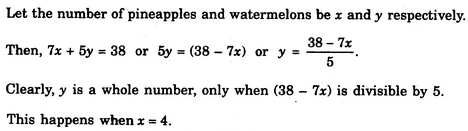# Verbal Reasoning - Arithmetic Reasoning

Exercise : Arithmetic Reasoning - Section 1
6.
A pineapple costs Rs. 7 each. A watermelon costs Rs. 5 each. X spends Rs. 38 on these fruits. The number of pineapples purchased is
2
3
4
Explanation:7.
A woman says, "If you reverse my own age, the figures represent my husband's age. He is, of course, senior to me and the difference between our ages is one-eleventh of their sum." The woman's age is
23 years
34 years
45 years
None of these
Explanation:

Let x and y be the ten's and unit's digits respectively of the numeral denoting the woman's age.

Then, woman's age = (10X + y) years; husband's age = (10y + x) years.

Therefore (10y + x)- (10X + y) = (1/11) (10y + x + 10x + y)(9y-9x) = (1/11)(11y + 11x) = (x + y)10x = 8yx = (4/5)y

Clearly, y should be a single-digit multiple of 5, which is 5.

So, x = 4, y = 5.

Hence, woman's age = 10x + y = 45 years.

8.
A girl counted in the following way on the fingers of her left hand : She started by calling the thumb 1, the index finger 2, middle finger 3, ring finger 4, little finger 5 and then reversed direction calling the ring finger 6, middle finger 7 and so on. She counted upto 1994. She ended counting on which finger ?
Thumb
Index finger
Middle finger
Ring finger
Explanation:

Clearly, while counting, the numbers associated to the thumb will be : 1, 9,17, 25,.....

i.e. numbers of the form (8n + 1).

Since 1994 = 249 x 8 + 2, so 1993 shall correspond to the thumb and 1994 to the index finger.

9.
A man has Rs. 480 in the denominations of one-rupee notes, five-rupee notes and ten-rupee notes. The number of notes of each denomination is equal. What is the total number of notes that he has ?
45
60
75
90
Explanation:

Let number of notes of each denomination be x.

Then, x + 5x + 10x = 48016x = 480x = 30.

Hence, total number of notes = 3x = 90.

10.
What is the product of all the numbers in the dial of a telephone ?
1,58,480
1,59,450
1,59,480
None of these